Publication date: 04/12/2021

## Control Limits for U Charts and C Charts

The lower and upper control limits, LCL, and UCL, are computed using the following formulas:

U chart LCL =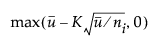U chart UCL =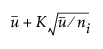C chart LCL =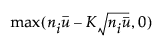C chart UCL =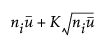The limits vary with ni.

ui is the number of nonconformities per unit in the ith subgroup. In general, ui = ci/ni.

K is the sigma multiplier and is set to 3 by default

ci is the total number of nonconformities in the ith subgroup

ni is the number of inspection units in the ith subgroup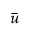is the average number of nonconformities per unit taken across subgroups. The quantityis computed as a weighted average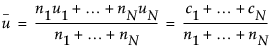N is the number of subgroups

Want more information? Have questions? Get answers in the JMP User Community (community.jmp.com).